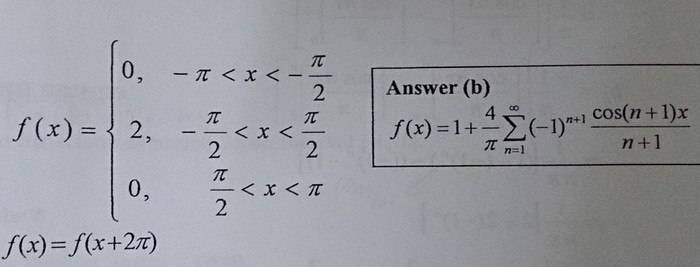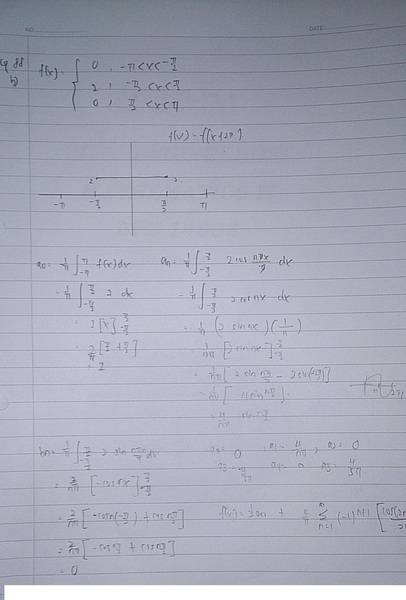# Fourier series

## Homework Statement

the answer that i get is different with the answer provided , is my answer wrong ? i got ( cos(2n -1) / 2n-1 )instead of ( cos(n+1) / n+1 )## The Attempt at a Solution

[/B]

Last edited by a moderator:

vela
Staff Emeritus
Homework Helper
It's easy enough to check yourself. Try plotting each series. You only need 4 or 5 terms each.

It's easy enough to check yourself. Try plotting each series. You only need 4 or 5 terms each.

I am not interested in the graph, I just wanna know the final answer....

Samy_A
Homework Helper
I am not interested in the graph, I just wanna know the final answer....
I actually followed vela's good advice, and the result is telling ...

Another test you could try: what happens with the given solution (answer b) when x=π?

Last edited:
I actually followed vela's good advice, and the result is telling ...

Another test you could try: what happens with the given solution (answer b) when x=π?
Cos(n +1 ) pi= (-1)^n.... What can we conclude from that??

Samy_A
Homework Helper
Cos(n +1 ) pi= (-1)^n.... What can we conclude from that??
No, that is not correct.
##\cos 2 \pi = \cos (1+1) \pi \neq {(-1)}^1##

Once you have the correct values, plug them in into the Fourier series of answer (b).

No, that is not correct.
##\cos 2 \pi = \cos (1+1) \pi \neq {(-1)}^1##

Once you have the correct values, plug them in into the Fourier series of answer (b).
It's (-1) ^ (n +1)

Samy_A